Python | Pandas TimedeltaIndex.difference

• Last Updated : 21 Sep, 2021

Python is a great language for doing data analysis, primarily because of the fantastic ecosystem of data-centric python packages. Pandas is one of those packages and makes importing and analyzing data much easier.
Pandas TimedeltaIndex.difference() function return a new Index with elements from the index that are not in other. This is the set difference of two Index objects. It’s sorted if sorting is possible.

Syntax : TimedeltaIndex.difference(other)
Parameters :
other : Index or array-like
Return : difference : Index

Example #1: Use TimedeltaIndex.difference() function to find the set difference of two TimedeltaIndex objects.

Python3

 # importing pandas as pdimport pandas as pd # Create the first TimedeltaIndex objecttidx1 = pd.TimedeltaIndex(data =['06:05:01.000030', '+23:59:59.999999',                                 '22 day 2 min 3us 10ns', '+23:29:59.999999',                                                         '+12:19:59.999999']) # Create the second TimedeltaIndex objecttidx2 = pd.TimedeltaIndex(data =['09:11:18.000030', '+23:59:59.999999',                                 '9 day 18 min 3us ', '+23:29:59.999999',                                                     '+12:19:59.999999']) # Print the first and second TimedeltaIndex objectprint(tidx1, "\n", tidx2)

Output :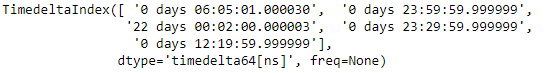Now we will use the TimedeltaIndex.difference() function to find the set difference.

Python3

 # find the set differencetidx1.difference(tidx2)

Output :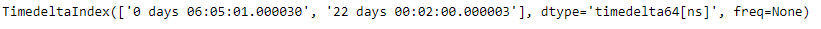As we can see in the output, the TimedeltaIndex.difference() function has returned a new object which contains only those elements which are unique to tidx1.

Example #2: Use TimedeltaIndex.difference() function to find the set difference of two TimedeltaIndex objects.

Python3

 # importing pandas as pdimport pandas as pd # Create the first TimedeltaIndex objecttidx1 = pd.TimedeltaIndex(start ='1 days 02:00:12.001124', periods = 5,                                              freq ='D', name ='Koala') # Create the second TimedeltaIndex objecttidx2 = pd.TimedeltaIndex(start ='3 days 02:00:12.001124', periods = 5,                                              freq ='D', name ='Koala') # Print the first and second TimedeltaIndex objectprint(tidx1, "\n", tidx2)

Output :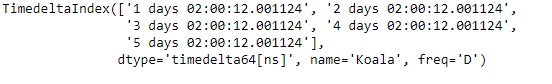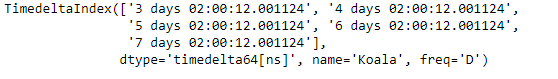Now we will use the TimedeltaIndex.difference() function to find the set difference.

Python3

 # find the set differencetidx1.difference(tidx2)

Output :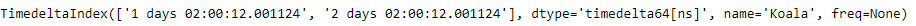As we can see in the output, the TimedeltaIndex.difference() function has returned a new object which contains only those elements which are unique to tidx1.

My Personal Notes arrow_drop_up